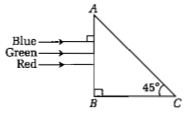Match the corresponding entries of Column 1 with Column 2. [Where m is the magnification produced by the mirror]

Column 1                         Column 2
A. m=-2                     a. Convex mirror
B. m=-1/2                  b. Concave mirror
C. m=+2                    c. Real image
D. m=+1/2                 d. Virtual Image

(a)A->a and c;B->a and d; C->a and b; D->c and d
(b)A->a and d; B->b and c; C->b and d; D-> b and c
(c)A->c and d; B->b and d;C->b and c;D->a and d
(d)A->b and c; B->b and c; C->b and d; D->a and d

Concept Questions :-

Reflection at spherical surface
High Yielding Test Series + Question Bank - NEET 2020

Difficulty Level:

The angle of incidence for a ray of light at a refracting surface of a prism is 45°. The angle of prism is 60°. If the ray suffers minimum deviation through the prism, the angle of deviation and refracting index of the material of the prism respectively are

(a)30°,$\sqrt{2}$

(b)45°,$\sqrt{2}$

(c)30°,$\frac{1}{\sqrt{2}}$

(d)45°,$\frac{1}{\sqrt{2}}$

Concept Questions :-

Prisms
High Yielding Test Series + Question Bank - NEET 2020

Difficulty Level:

Concept Questions :-

Prisms
High Yielding Test Series + Question Bank - NEET 2020

Difficulty Level:

In an astronomical telescope in normal adjustment a straight black line of length L is drawn on inside part of the objective lens. The eye-piece forms a real image of this line. The length of this image is l. The magnification of the telescope is:

(a)L/l+1

(b)L/l-1

(c)L+1/L-1

(d)L/l

Concept Questions :-

Telescope
High Yielding Test Series + Question Bank - NEET 2020

Difficulty Level:

A beam of light consisting of red, green and blue colours is incident on a right angled prism. The refractive index of the material of the prism for the above red, green and blue wavelengths are 1.39, 1.44 and 1.47, respectively.The prism will

(a) separate the blue colour part from the red and green colours

(b) separate all the three colours from one another

(c) not separate the three colours at all

(d) separate the red colour part from the green and blue colours

Concept Questions :-

Total internal reflection
High Yielding Test Series + Question Bank - NEET 2020

Difficulty Level:

If the focal length of the objective lens is increased, then magnifying power of :

(a) microscope will increase but that of telescope decrease

(b) microscope and telescope both will increase

(c) microscope and telescope both will decrease

(d) microscope will decrease but that of telescope will increase

Concept Questions :-

Telescope
High Yielding Test Series + Question Bank - NEET 2020

Difficulty Level:

The angle of a prism is A. One of its refracting surfaces is silvered. Light rays falling at an angle of incidence 2A on the first surface returns back through the same path after suffering reflection at the silvered surface. The refractive index μ of the prism is

(a)2sinA

(b)2cosA

(c)1/2cosA

(d)tanA

Concept Questions :-

Prisms
High Yielding Test Series + Question Bank - NEET 2020

Difficulty Level:

A plane-convex lens fits exactly into a plano-concave lens. Their plane surfaces are parallel to each other. If lenses are made of different materials of refractive indices in  μ1 and μ2 and R is the radius of curvature of the curved surface of the lenses. then the focal length of the combination is

(a) R/2(μ1+μ2)

(b) R/2(μ1-μ2)

(c) R/(μ1-μ2)

(d) 2R/(μ2-μ1)

Concept Questions :-

Lensmakers' formula
High Yielding Test Series + Question Bank - NEET 2020

Difficulty Level:

For a normal eye, the cornea of eye provides a converging power of 40 D and the least converging power of the eye lens behind the cornea is 20 D. Using this information, the distance between the retina and the cornea-eye lens can be estimated to be

(a) 5 cm

(b) 25 cm

(c) 1.67 cm

(d) 1.5 cm

Concept Questions :-

Human eye
High Yielding Test Series + Question Bank - NEET 2020

Difficulty Level:

When a biconvex lens of glass having refractive index 1.47 is dipped in a liquid, it acts as a plane sheet of glass. This implies that the liquid must have refractive index

(a)equal to that of glass

(b)less than one

(c)greater than that of glass

(d)less than that of glass

Concept Questions :-

Lenses